#devoworm-group /Devolearn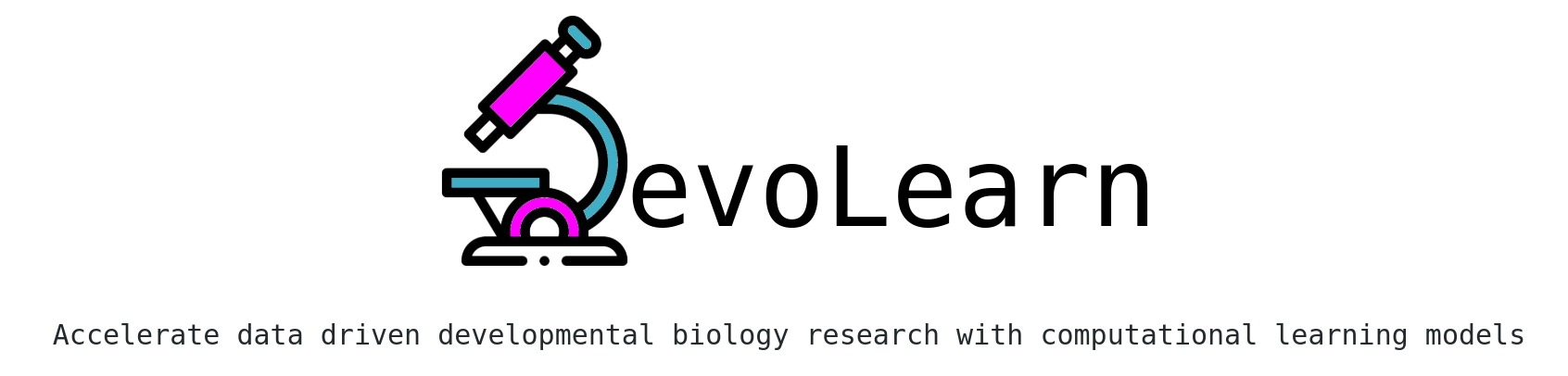## Contents

### Installation

pip install devolearn


### Example notebooks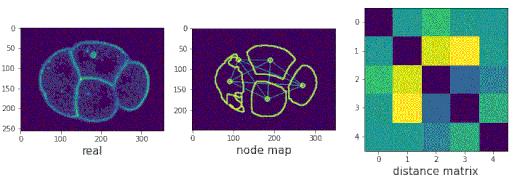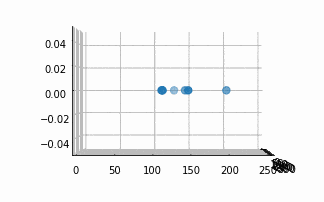### Segmenting the Cell Membrane in C. elegans embryo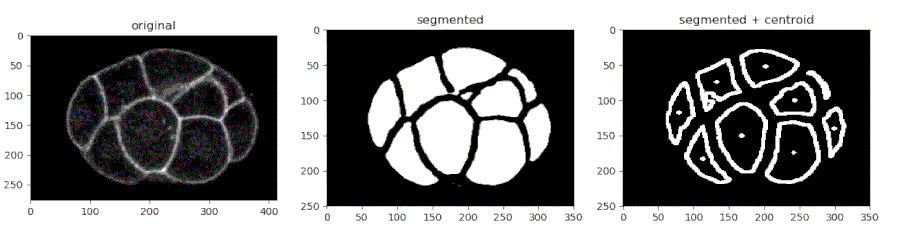• Importing the model
from devolearn import cell_membrane_segmentor
segmentor = cell_membrane_segmentor()

• Running the model on an image and viewing the prediction
seg_pred = segmentor.predict(image_path = "sample_data/images/seg_sample.jpg")
plt.imshow(seg_pred)
plt.show()

• Running the model on a video and saving the predictions into a folder
filenames = segmentor.predict_from_video(video_path = "sample_data/videos/seg_sample.mov", centroid_mode = False, save_folder = "preds")

• Finding the centroids of the segmented features
seg_pred, centroids = segmentor.predict(image_path = "sample_data/images/seg_sample.jpg", centroid_mode = True)
plt.imshow(seg_pred)
plt.show()

• Saving the centroids from each frame into a CSV
df = segmentor.predict_from_video(video_path = "sample_data/videos/seg_sample.mov", centroid_mode = True, save_folder = "preds")
df.to_csv("centroids.csv")


### Segmenting the Cell Nucleus in C. elegans embryo• Importing the model
from devolearn import cell_nucleus_segmentor
segmentor = cell_nucleus_segmentor()

• Running the model on an image and viewing the prediction
seg_pred = segmentor.predict(image_path = "sample_data/images/nucleus_seg_sample.jpg")
plt.imshow(seg_pred)
plt.show()


### Generating synthetic images of embryos with a Pre-trained GAN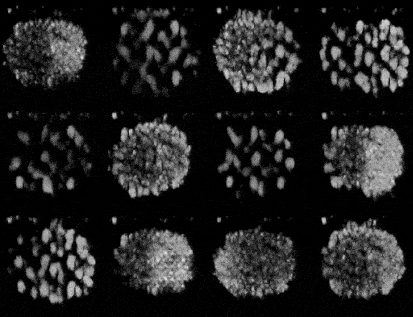• Importing the model
from devolearn import Generator, embryo_generator_model
generator = embryo_generator_model()

• Generating a picture and viewing it with matplotlib
gen_image = generator.generate()
plt.imshow(gen_image)
plt.show()

• Generating n images and saving them into foldername with a custom size
generator.generate_n_images(n = 5, foldername= "generated_images", image_size= (700,500))


### Predicting populations of cells within the C. elegans embryo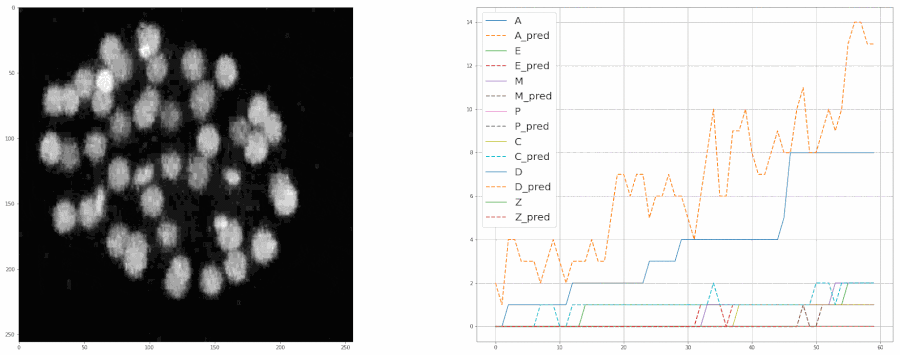• Importing the population model for inferences
from devolearn import lineage_population_model

• Loading a model instance to be used to estimate lineage populations of embryos from videos/photos.
model = lineage_population_model(device = "cpu")

• Making a prediction from an image
print(model.predict(image_path = "sample_data/images/embryo_sample.png"))

• Making predictions from a video and saving the predictions into a CSV file
results = model.predict_from_video(video_path = "sample_data/videos/embryo_timelapse.mov", save_csv = True, csv_name = "video_preds.csv", ignore_first_n_frames= 10, ignore_last_n_frames= 10, postprocess = False)

• Plotting the model's predictions from a video
plot = model.create_population_plot_from_video(video_path = "sample_data/videos/embryo_timelapse.mov", save_plot= True, plot_name= "plot.png", ignore_last_n_frames= 0, postprocess = False)
plot.show()


Model Data source
Segmenting the cell membrane in C. elegans embryo 3DMMS: robust 3D Membrane Morphological Segmentation of C. elegans embryo
Segmenting the nucleus in C. elegans embryo C. elegans Cell-Tracking-Challenge dataset
Cell lineage population prediction + embryo GAN EPIC dataset

Model Huggingface
Segmenting the cell membrane in C. elegans embryo Cell Membrane segmentor
Segmenting the nucleus in C. elegans embryo C. elegans Nucleus segmentor
Cell lineage population prediction Lineage population